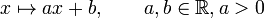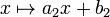# GAPlus(1,R) is rationally powered

This article gives the statement, and possibly proof, of a particular group or type of group (namely, GAPlus(1,R) (?)) satisfying a particular group property (namely, Rationally powered group (?)).

## Statement

The group$GA^+(1,\R)$, denoted GAPlus(1,R), defined explicitly as the group under composition of maps from$\R$ to$\R$ of the form:$x \mapsto ax + b, \qquad a,b \in \R, a > 0$

is a rationally powered group. In other words, for any positive integer$n$ and any element$u \in GA^+(1,\R)$, there is a unique$v \in GA^+(1,\R)$ such that$v^n = u$.

## Proof

Suppose$u$ is the map:$x \mapsto a_1x + b_1$

We want to find all elements$v$ that are maps of the form$x \mapsto a_2x +b_2$ such that$v^n = u$. By composing$v$ with itself$n$ times, we get$v^n = x \mapsto a_2^nx + b_2(1 + a_2 + \dots + a_2^{n-1})$

For this to equal$u$, we know that the coefficient of$x$ and the constant term should match up separately, so we get:$a_1 = a_2^n$

and:$b_1 = b_2(1 + a_2 + \dots + a_2^{n-1})$

Solving, we get that the unique solution is the element$v$ with:$a_2 = a_1^{1/n}, \qquad b_2 = \frac{b_1}{1 + a_1^{1/n} + \dots + a_1^{(n-1)/n}}$

In other words, the solution is:$x \mapsto a_1^{1/n}x + \frac{b_1}{1 + a_1^{1/n} + \dots + a_1^{(n-1)/n}}$